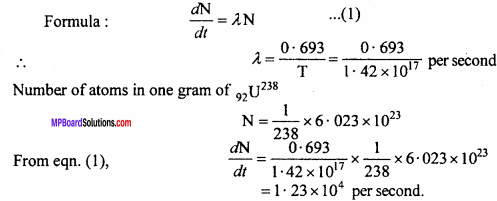# MP Board Class 12th Physics Important Questions Chapter 13 Nuclei

## MP Board Class 12th Physics Important Questions Chapter 13 Nuclei

### Nuclei Important Questions

Nuclei Objective Type Questions

Question 1.
Choose the correct answer of the following:

Half-life formula is the time required for the amount of something to fall to half its initial value.

Question 1.
The relation between half life time Tm and decay constant of a radioactive material is: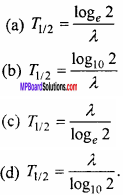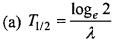Question 2.
The decayed part of a radioactive sample in two half lives will be :
(a) One fourth
(b) Half
(c) Three fourth
(d) Full part
(c) Three fourth

Question 3.
The α – particle is a :
(a) Hydrogen nucleus
(b) Deuterium nucleus
(c) Helium nucleus
(d) Tritium.
(c) Helium nucleusQuestion 4.
The value of A and Z in the nuclear reaction $$_{ 238 }^{ 92 }{ U }$$ → $$_{ A }^{ Z }{ Th }$$ + $$_{ 4 }^{ 2}{ He }$$ will be :
(a) 234,94
(b) 234,90
(c) 238,94
(d) 238,90.
(b) 234,90

Question 5.
The source of energy in the sun is :
(a) Nuclear fission
(b) Nuclear fusion
(c) Chemical reaction
(d) Photo electric reaction.
(b) Nuclear fusion

Question 6.
For the nuclear fusion which of the following is suitable :
(a) Heavy nucleus
(b) Lighter nucleus
(c) Atom bomb
(b) Lighter nucleus

Question 7.
In the symbol of nucleus $$_{ Z }^{ A }{ X }$$ there are :
(a) Z – neutrons, (A – Z) protons
(b) Z – protons, (A – Z) neutrons
(c) Z – protons, A neutrons
(d) A protons. Z neutrons.
(b) Z – protons, (A – Z) neutrons

Question 8.
In the gamma ray emission of a nucleus :
(a) Only proton number varies
(b) Number of neutrons and protons both varies
(c) There is no change in number of protons and neutrons
(d) Only number of neutrons varies.
(c) There is no change in number of protons and neutrons

Question 2.
Fill in the blanks :

1. In the controlled chain reaction the fast moving neutrons are slowed down by using moderator. These neutrons are called …………..
2. The Einstein’s mass energy equivalence relation is …………..
3. The unstable nucleus obtained by artificial radioactivity is called …………..
4. The mass of neutrons in a nucleus is nearly equal to mass of …………..
5. In the process of ………….. the lighter nuclei together makes a heavy nucleus.
6. In nuclear reactor the heavy water is used as …………..
7. The SI unit of radio activity is …………..
8. In the range mass number A = 30 to A = 170 the value of ………….. is nearly constant.

1. Thermal neutrons
2. E = me2
4. Protons
5. Fusion
6. Moderator
7. Becquerel (Bq)
8. Per nucleon binding energy.

Question 3.
Match the columns :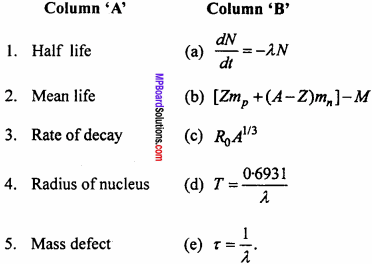1. (d)
2. (e)
3. (a)
4. (c)
5. (b)

Question 4.
Write the answer in one word/sentence :

1. Write the equation showing the decay of free neutron.
2. The energy distribution of P – rays is continuous. Why?
3. In the α and β particles, which one has more ionization power?
4. The ionization power of α – particles is high. Why?
5. The penetrating power of γ(gamma) – rays is very high. Why?
6. Write an equation showing nuclear fusion.
7. Which part of the electromagnetic spectrum has highest penetrating power?
8. What is order of size of nucleus?
9. Does the density of all nuclei remain same? If yes then write its order.
10. How is nuclear radius related to atomic mass?

1. 0n11H1 + -1B0 + $$\overline { ν }$$
2. Because during the β – decay anti – neutrino is emitted
3. α – particles
4. Because α – particles have large mass and large size and their velocity is low
5. Because their velocity is very high and they do not have any charge,
6. 1H2 + 1H22He4 + Q
7. γ – rays
8. 10-15 m
9. Yes, 1017 per cubic metre
10. R = R0 A1/3

Nuclei Very Short Answer Type Questions

Question 1.
What will be the ratio of radii of two nucleus of mass number A1 and A2?
Solution:
From the formula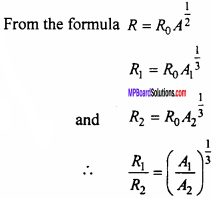Question 2.
What will be the energy equivalent to 10 milligram?
Solution:
E = mc2
= 10 x 10-6 x (3 x 108)2
= 9 x 109 joule.

Question 3.
What are thermal neutrons?
In the process of controlled nuclear fission, the fast moving neutrons are slowed down with the help of moderation. These slow stray neutrons are called as thermal neutrons.

Question 4.
Does the ratio of neutron and proton after emission of α – particle in a nucleus increase, decrease or remain constant?
The ratio can increase, decrease or can remain same. It depends upon the nature of nucleus.Question 5.
Nuclear fusion is not possible in laboratory. Why?
For nuclear fusion very high temperature (≈ 107K) and very high pressure is required which is not possible in laboratory.

Question 6.
Is a free neutron a stable particle?
No, free neutron is decayed in a proton, an electron and an antineutrino ($$\overline { ν }$$).
0n11P1 + -1e0 + $$\overline { ν }$$

Question 7.
In heavy nucleus the number of neutrons is more than number of protons. Explain why?
In a nucleus there is a coulomb’s repulsive force between protons in addition to a strong attraction nuclear force. On the other hand in case of neutrons, within the nucleus, there is only a short range attractive nuclear force. For heavy nuclei to be stable the repulsive force must be less. This is possible only if the number of neutrons is more than number of protons.

Question 8.
If a radioactive substance that is capable to emit a, p and y rays is kept on a piece of paper, then which rays has the maximum possibility to be stoped?
As the mass of α – particles is more and its penetrating power is least, so it will be stopped.

Question 9.
Why is heavy water used as moderator in a nuclear reactor?
Heavy water contains protons (of mass nearly equal to the mass of neutrons). Fast moving neutrons undergo elastic collision with these slow moving neutrons and thus get slowed down. Hence heavy water is used as moderator.Question 10.
An atom expressed by YXA emits n α – particles. How many proton will remain in it? What will be the new atomic number of new atom?
In an α – particles there are 2 protons. If n α – particles are emitted then 2n proton will be reduced.
∴ No. of remaining protons = Z – 2n
Atomic number = Y – 2n.

Question 11.
Penetrating power of β – particles is more than that of α – particles but its penetrating power is less. Why?
β – particles have small size and high velocity so its penetrating power is more than α – particles. Due to small size and high velocity the probability of collision with gas molecules decreases and hence ionization power becomes less.

Question 12.
The nuclear fusion could not be used as an experimental and controlled source of energy till now. Why?
The nuclear fusion takes place at very high temperature. Due to such very high temperature it is not possible to control it.

Question 13.
Why is a neutron preferred as a bombarding particle in nuclear fission?
Neutron is a neutral particle i.e., it has no charge. So it is neither repelled nor attracted by the nucleus and hence can penetrate deep into the nucleus to cause a nuclear reaction.

Question 14.
β – rays are emitted from nucleus. They are made of fast moving electron though there are no electrons in the nucleus. Why it is so?
Nucleus has no electron in it. Actually due to decay of neutron into proton and electron, the β – rays (particle) are produced.Question 15.
Justify that gamma rays have more penetrating power and α – particles have more ionizing power.
Gamma rays are electromagnetic waves. Its speed is equal to the speed of light. So they can penetrate deeply into the matter i.e., their penetrating power is high, α – particles has high mass and posses greater kinetic energy. So when they collide with any atom, it transfer its energy to orbital electrons and they are ejected. Hence α – particles has high ionizing power.Question 16.
The mass number of two nucleus are 1 : 2. What is the ratio of their nuclear density?
1 : 1 because nuclear density does not depend on mass number.

Question 17.
What is effect of temperature and pressure or radio activity?
No, effect.

Question 18.
Due to the emission of α – particles does the ratio of neutron to proton decrease, increase or remains the same?
The ratio $$\frac {n}{p}$$ increases, because number of protons decreases by 2.

Question 1.
Which particles remains inside the nucleus? What will be the number of neutrons in the nucleus zXA?
The neutrons and protons remains inside the nucleus. In the nucleus zXA number of neutrons be A – Z.

Question 2.
Define decay constant and mean life of a radioactive substance write their units.
Decay Constant:
The decay constant is defined as the reciprocal of that time interval during which the number of active nuclei in a given sample of a radioactive substance reduces to times of the initial value of number of nuclei.
If T is half life time and X is decay constant then
T = $$\frac {0.6931}{λ}$$
Its unit is “per second”.

Mean life:
The reciprocal of decay constant is called mean life, its unit is ‘second’.Question 3.
What is radioactivity? Write the names of the rays emitted from radio – active substances.
Radioactivity is that property by virtue of which the nucleus of a heavy element disintegrates itself with the emission of radiation without being forced by any external agent to do so.

Following rays are emitted from a radioactive substance :

1. α – rays
2. β – rays
3. γ – rays.

Question 4.
A radioactive nucleus decays as given below :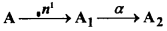If the mass number of A2 is 176 and atomic number is x then what is the atomic number and mass number of A1 and A? Are they isotopes or isobars?
The mass number of A2 = 176,
atomic number of A2 = 71.
Due to decay of α – particle mass number decreases by 4 and atomic number decreases by 2.
∴ Mass number of A1 = 176 + 4 = 180 and
its atomic number = 71 + 2 = 73
Similarly
The mass number of A = 180 + 1 = 181 and
atomic number of A = 73 + 0 = 73
Since, the atomic number of A and A1 are same so they are isotopes.

Question 5.
Define decay constant of a radioactive substance. Write its relation with half life?
The decay constant is defined as the reciprocal of that time interval during which the number of active nuclei in a given sample of a radioactive substance reduces to times of the initial value of number of nuclei.
If T is half life time and X is decay constant then
T = $$\frac {0.6931}{λ}$$.Question 6.
What is nuclear fission? Give an example.
When a heavier nucleus is bombarded with neutrons then it get splitted into two lighter nuclei. This process is called as nuclear fission.
A huge amount of energy is released in this process.
92U235 + 0n156Ba141 + 36kr92 + 3 0n1 + 200 MeV.

Question 7.
What is nuclear fusion? Give an example.
When two lighter nuclei fused together to form a heavier nucleus then a huge amount of energy is released in this process. This process is called nuclear fusion.
1H2 + 1H22He4+ 24MeV.

Question 8.
A radioactive element with mass number 218 and atomic number 84 emits a β – particle. What would be the mass number and atomic number after decay?
Since, after the emission of β – particle there is no change in the mass number of element but its atomic number is increased by 1.
Therefore the mass number will be 218 and atomic number will be 84 + 1 = 85.

Question 9.
The ratio of radii of two nuclei are 1 : 2. Find out the ratio of their mass number.
Solution:Question 10.
The nuclear fusion process is difficult as compared to nuclear fission. Why?
For nuclear fusion a high temperature (≈ 107 K) is required. It is very difficult to achieve this temperature, so nuclear fusion is difficult.

Question 11.
Write the important properties of nuclear forces.

1. Nuclear forces are attraction force
2. These forces does not depend on charge
3. These forces are short range forces
4. They are strong forces
5. The nuclear forces are not central forces.

Question 12.
Write properties of nuclear forces. Prove that the density of nucleus is independent of mass number.
Properties of nuclear forces :

1. Nuclear forces are attraction force
2. These forces does not depend on charge
3. These forces are short range forces
4. They are strong forces
5. The nuclear forces are not central forces.

Let the mass number of a nucleus is A and radius is r then volume of nucleus beThus, it is clear that material density of a nucleus is independent of its mass number.Question 13.
Write difference between nuclear fusion and nuclear fission.
Difference between nuclear fusion and nuclear fission :
Nuclear fission:

• In this prosses a heavy nucleus splitted into two lighter nuclei.
• This process is possible at normal temperature.
• Per fission released energy is very high (200 MeV)

Nuclear fusion:

• In this process two lighter nuclei fused together to form a heavy nucleus.
• This process is possible at very high temperature.
• Per fusion released energy is comparatively less (24 MeV).

Question 1.
Define ‘electron volt’ and ‘Atomic mass unit”. Find out the energy equivalent to mass of a proton in joule.
Electron volt:
The energy acquired by an electron when it is being accelerated by a potential difference of 1 volt is called ‘electron volt’.
1 electron volt = 1.6 x 10-19J.

Atomic mass unit:
An atomic mass unit is defined as ($$\frac {1}{12}$$) of the mass of 1 atom of carbon – 12.
1 atomic mass unit = 1.66 x 10-27kg
(1 a.m.u. or only 1u)
The mass of proton = 1.673 x 10-27 x (3 x 108 )2
= 15.05 x 10-11J
= 1.505 x 10-10J
∴ The energy equivalent mass of 1 proton is 1.505 x 10-10JQuestion 2.
Draw the graph showing the variation of binding energy per nucleon with the mass number. Write important conclusions drawn from it. (CBSE 1994)
Or
Draw the graph showing the variation of binding energy per nucleon with mass number. Highlight the region where the nuclei are more stable. (CBSE 1996)
Or
Draw the graph showing the variation of binding energy per nucleon with the mass number. Highlight the region where the nuclear fusion takes place. (CBSE 1996)
From the binding energy curve, it follows that:

1. The average binding energy/ nucleon for nearly all elements is 8 MeV

2. The maximum value of binding energy per nucleon is 8.79 MeV and it is for Fe. This explains the large abundance of Fe in nature

3. Since for nuclei of intermediate mass numbers, the value of the binding energy per nucleon is around the maximum value, these nuclei are the most stable

4. The binding energy/ nucleon decreases for nuclei of mass number more than 56 and its least value is 7.6 MeV.5. Below mass number 28, there are peaks in the curve corresponding to those nuclei whose mass numbers are multiples of four. These nuclei contain an equal number of protons and neutrons. Though peaks occur corresponding to $$_{ 4 }^{ 2 }{ He}$$, $$_{ 8 }^{ 4 }{ Be }$$, $$_{ 12 }^{ 6 }{ C }$$, $$_{ 16 }^{ 8 }{ O }$$ and $$_{ 20}^{ 10 }{ Ne }$$, we have shown only the first peak corresponding to $$_{ 4 }^{ 2 }{ He }$$ Obviously, these nuclei have more binding energy per nucleon than their neighbours. This explains the stability of an α – particle ($$_{ 4 }^{ 2 }{ U }$$)

6. The smallest value of the binding energy per nucleon is in the case of a deuteron ($$_{ 2 }^{ 1 }{ U }$$) and its value (2.2 MeV)/2 = 1.1 MeV. This is confirmed by the fact that a photon whose energy is 2.2 MeV or more, can split a deuteron into a free neutron and a free proton. This phenomenon is called the photodisintegration of a deuteron.

Question 3.
Calculate binding energy per nucleon of 26Fe56.
Given : m($$_{ 26 }^{ 56 }{ Fe }$$) = 55.934939 a.m.u., m (proton ) = 1.007825 a.m.u., m (neutron) = 1.008665 a.m.u. (CBSE 1993,95, 2000 Supp.)
Solution:
Number of protons in = $$_{ 26 }^{ 56 }{ He }$$ is 26 and number of neutrons is 56 – 26 = 30.
∴ Mass of 26 protons = 1 0077825 x 26
= 26.202345 a.m.u.
Mass of 30 neutrons = 1.008665 x 30
= 30.25995 a.m.u.
Total mass of nucleons = 26.202345 + 30.25995
= 56.4623 a.m.u.
Mass of 26Fe56 = 55.934939.a.m.u.,
∴ Mass defect ∆m = 56.4623 – 55.934939
= 0.527361 a. m. u.
∴ Binding energy of 26Fe56 = 0.527361 x 931
= 490.973091 MeV
Binding energy per nucleon = $$\frac {490.973091}{56}$$
= 8.7674 MeV.Question 4.
Define the term half-life period and decay constant of a radioactive sample. Derive a relation between these terms.
The half – life of a radioactive substance is the time it takes half of a given number of radioactive nuclei to decay. (CBSE 1995, 2001)
The SI unit of Tm is second (s).
Example:
Half life of radium is 1600 years. It means that if 1 gm of radium is taken then after 1600 years $$\frac {1}{2}$$ gm of radium will decay.
Let us assume that at time t = 0, the number of radioactive nuclei present is N0 and after time t, the number of radioactive nucleus remaining is N, then according to law of radioactive decay,
N = N0e-λt
Where, λ is decay constant.
If the half – life of radioactive sample is T1/2, then at t = T1/2
N = $$\frac { { N }_{ 0 } }{ 2 }$$
Substituting in equation (1), we get ,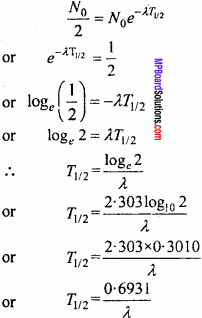From equation (2), it is clear that half life of a radioactive sample is inversely proportional to decay constant.

Question 5.
State law of radioactive decay and derive the following formula :
N = N0 e-λt, where symbols have their usual meaning.
According to the radioactive decay law :
The rate at which a particular decay process occurs in a radioactive sample is proportional to the number of radioactive nuclei present (that is, those nuclei that have not yet decayed).
Let N0 = Total number of radioactive nuclei present originally at time t = 0,
(t = 0 refers to the time when the radioactive element is freshly separated from its by products), and N = Total number of radioactive nuclei present at any time t.According to the radioactive decay law,
– $$\frac {dN}{dt}$$ ∝ N
Thus, – $$\frac {dN}{dt}$$ = λN
Where A is a constant of proportionality and is called the disintegration constant or decay constant.
Equation (1) can be written as
$$\frac {dN}{dt}$$ = – λdt
When t = 0, N = N0 and when t = t,N = N.
Integrating equation (2) within proper limits,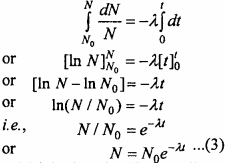From equation (3) it is clear that (i) the radioactive decay is an exponential process i.e., the decay is fast initially and later on it becomes slow.

Nuclei Numerical Questions

Question 1.
An imaginary fission reaction is given below :
$$_{ 92 }^{ 236 }{ X }$$ → $$_{ a}^{ 141 }{ Y }$$ + $$_{ 36 }^{ b }{ Z }$$ + 3$$_{ 0 }^{ 1 }{ n }$$
Find out value of a and b.
Solution:
a + 36 + 0 = 92
a = 56.
and 141 + b + 3 = 236
b = 92.Question 2.
The half life of a radioactive substance is 30 days. What will be the number of radioactive atoms after 90 days?
Solution:
Given : T = 30 days, t = 90 days
n = $$\frac {t}{T}$$ = $$\frac {90}{30}$$ = 3
Formula : N = N0
= N0($$\frac {1}{2}$$)n
= N0($$\frac {1}{2}$$)3
= $$\frac { { N }_{ 0 } }{ 8 }$$
Thus, the number of radioactive atoms be $$\frac {1}{8}$$ times of their initial value.

Question 3.
How many fission per second takes place if its half life for α – decay is 1.42 x 1017sec?
Solution: